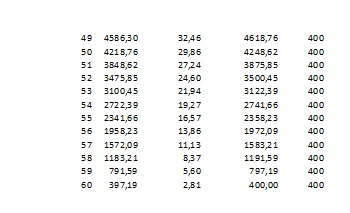## Uniform serie present-worth factor — Calculating interest rate

In the MSE question Calculating interest rate of car financing, Juan Hynix asks:

I want a new car which costs $26.000. But there’s an offer to finance the car: Immediate prepayment: 25% of the original price The amount left is financed with a loan: Duration: 5 years, installment of$400 at the end of every month.

So I need to calculate the rate of interest of this loan. Do I need Excel for this exercise? Or which formula could I use for this exercise?

You could use Excel (see below) or you could solve the equation$(2)$ below numerically, e.g. using the secant method.

We have a so called uniform series of$n=60$ constant  installments$m=400$.Let$i$ be the nominal annual interest rate. The interest is compounded monthly, which means that the number of compounding periods per year is$12$. Consequently, the monthly installments$m$ are compounded at the interest rate per month$i/12$. The value of$m$ in the month$k$ is equivalent to the present value$m/(1+i/12)^{k}$. Summing in$k$, from$1$ to$n$, we get a sum that should be equal to$P=26000-\dfrac{26000}{4}=19500.$

This sum is the sum of a geometric progression of$n$ terms, with ratio$1+i/12$ and first term$m/(1+i/12)$. So$P=\displaystyle\sum_{k=1}^{n}\dfrac{m}{\left( 1+\dfrac{i}{12}\right) ^{k}}=\dfrac{m}{1+\dfrac{i}{12}}\dfrac{\left( \dfrac{1}{1+i/12}\right) ^{n}-1}{\dfrac{1}{1+i/12}-1}=m\dfrac{\left( 1+\dfrac{i}{12}\right) ^{n}-1}{\dfrac{i}{12}\left( 1+\dfrac{i}{12}\right) ^{n}}.\qquad (1)$

The ratio$P/m$ is called the series present-worth factor (uniform series)$^1$.

For$P=19500$,$m=400$ and$n=5\times 12=60$ we have:$19500=400\dfrac{\left( 1+\dfrac{i}{12}\right) ^{60}-1}{\dfrac{i}{12}\left( 1+\dfrac{i}{12}\right) ^{60}}.\qquad (2)$

I solved  numerically$(2)$ for$i$ using SWP and got$i\approx 0.084923\approx 8.49\%.\qquad (3)$

ADDED. Computation in Excel for the principal$P=19500$ and interest rate$i=0.084923$ computed above. I used a Portuguese version, that’s why the decimal values show a comma instead of the decimal point.

– The Column$k$ is the month ($1\le k\le 60$).
– The 2nd. column is the amount$P_k$ still to be payed at the beginning of month$k$.
– The 3rd. column is the interest$P_ki/12$ due to month$k$.
– The 4th. column is the sum$P_k+P_ki/12$.
– The 5th column is the installment payed at the end of month$k$.

The amount$P_k$ satisfies$P_{k+1}=P_k+P_ki/12-m.$ We see that at the end of month$k=60$,$P_{60}+P_{60}i/12=400=m$. The last installment$m=400$ at the end of month$k=60$ balances entirely the remaining debt, which is also$400$. We could find$i$ by trial and error. Start with$i=0.01$  and let the spreadsheet compute the table values, until we have in the last row exactly$P_{60}+P_{60}i/12=400$.$^1$ James Riggs, David Bedworwth and Sabah Randdhava, Engineering Economics, McGraw-Hill, 4th. ed., 1996, p.43.